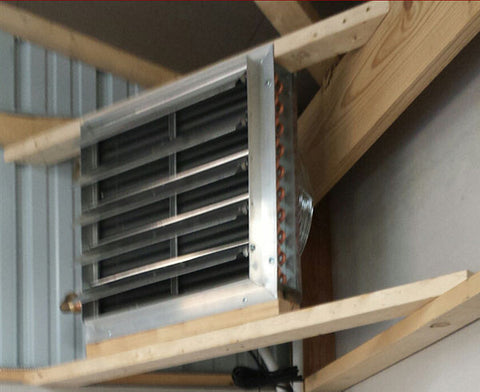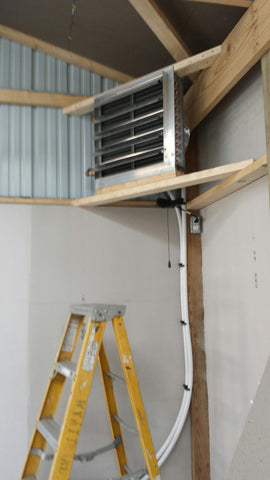# \$569.95 \$629.50

Unit heaters are used to heat garages, pole barns, workshops, and other spaces that are open. They are generally hung from the ceiling in a corner. These unit heaters have 3-Speed Quiet Fans, they are pre-wired with switch and cord, and the unit heater can be plugged into a thermostat for steady heat. (Thermostat sold separately.)

To calculate the size unit heater required for your space, use the following formula:

Calculate the cubic footage of the space to be heated by multiplying building length x width x ceiling height.  A 30 x 40 pole barn with 14 foot average ceilings* will have (30x40x14=) 16,800 cubic feet of space to heat.

Then multiply the cubic feet times the "insulation factor" to get BTUs required to properly heat the space.  Insulation factors are as follows:

4 - Excellent Insulation

5 - Average Insulation

7 - Poor Insulation

So if in this example, let's say you have average insulation and 16,800 cubic feet of space to heat. Multiply 16,800 times 5 equals 84,000. You must have one or more unit heaters that produce a total of 84,000 BTUs of heat.

Importantly, if you have a space that requires a large amount of heat, you may elect to install more than one unit heater to spread the heat around in the space better. For example, a workshop that needs  250,000 BTUs could be heated with THREE 90,000 BTU unit heaters. Just make sure that the sum of the unit heater BTU output is greater than the calculated BTU requirements.

For more details,